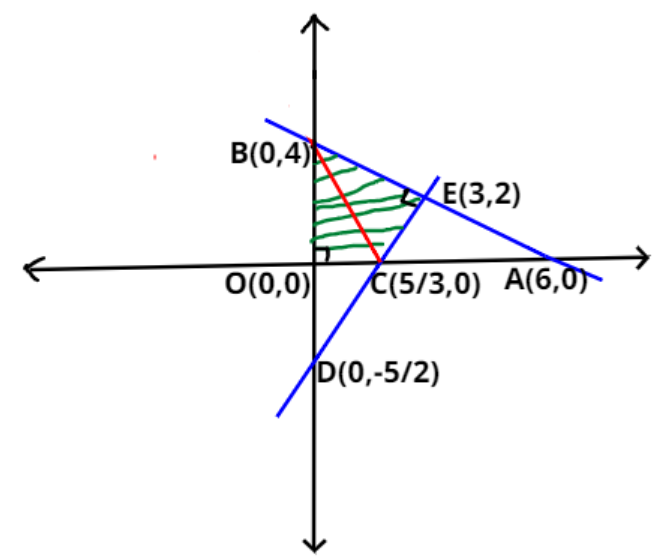QuestionAnswers

# The line $2x + 3y = 12$ meets the x-axis at A and y-axis at B. The line through $\left( {5,5} \right)$ perpendicular to AB meets the axes and the line AB at C, D, E respectively. If O is the origin of coordinates, then the area of OCEB is?Verified
149.7k+ views
Hint:- Use intercept form of line to get coordinates of points easily.As we are given with the line,
$\Rightarrow 2x + 3y = 12$ (1)
We know that, according to the intercept form of the equation. If any line is of form
$\Rightarrow \dfrac{x}{a} + \dfrac{y}{b} = 1$ (2)

Then it cuts off an intercept of a and b on x and y axis respectively
And the points where it cuts x and y axis are $\left( {a,0} \right)$ and $\left( {0,b} \right)$ respectively.
So, dividing both sides of equation 1 by 12. We get,
$\Rightarrow \dfrac{x}{6} + \dfrac{y}{4} = 1$ (3)
On comparing equation 3 with equation 2. We get, $a = 6$and $b = 4$.
So, line $2x + 3y = 12$cuts x-axis at $A \equiv \left( {6,0} \right)$ and y-axis at $B \equiv \left( {0,4} \right)$.
As, we know that slope of a line passing through two points $\left( {{x_1},{y_1}} \right)$ and $\left( {{x_2},{y_2}} \right)$is given as
$\Rightarrow m = \dfrac{{{y_2} - {y_1}}}{{{x_2} - {x_1}}}$
As line at equation 1 passes through points A and B.
So, slope of line $2x + 3y = 12$ will be $m = \dfrac{{4 - 0}}{{0 - 6}} = \dfrac{{ - 2}}{3}$
As we know that relation between slopes of two lines perpendicular to each other is given as, $\Rightarrow {m_1}{m_2} = - 1$
So, the slope of line perpendicular to line AB will be $\dfrac{{ - 1}}{m}$, where m is the slope of line AB.
So, the slope of the line perpendicular to AB will be $\dfrac{3}{2}$.
Now we had to find the equation of line perpendicular to line AB and passing through point $\left( {5,5} \right)$.
So, now using one-point form. Because we know the slope and point through which line passes.
Equation of line passing through $\left( {5,5} \right)$ and perpendicular to AB will be,
$\Rightarrow \left( {y - 5} \right) = \dfrac{3}{2}\left( {x - 5} \right)$
$\Rightarrow 3x - 2y = 5$ (4)
Dividing both sides of the above equation by 5. We get,
$\Rightarrow \dfrac{x}{{\left( {\dfrac{5}{3}} \right)}} + \dfrac{y}{{\left( {\dfrac{{ - 5}}{2}} \right)}} = 1$
On comparing the above equation with equation 2. We get,
$a = \dfrac{5}{3}$ and $b = \dfrac{{ - 5}}{2}$
So, line perpendicular to line AB and passing through point $\left( {5,5} \right)$ meets,
x-axis at $C \equiv \left( {\dfrac{5}{3},0} \right)$and y-axis at $D \equiv \left( {0,\dfrac{{ - 5}}{2}} \right)$. (see figure)
Now, solving equation 4 with equation 1. To get the coordinates of point E. (given in question)
Multiplying equation 1 by 2 and equation 4 by 3 and then adding we get,
$\Rightarrow 4x + 6y + 9x - 6y = 24 + 15 \\ \Rightarrow x = 3 \\$
Putting the value of x in equation 1. We get, $y = 2$
So, coordinates of point E will be $E \equiv \left( {3,2} \right)$.
As we all know that O is the origin. So, $O \equiv \left( {0,0} \right)$
Now, we had to find the area of the region OCEB.
As, we can see from the above figure that,
$\Rightarrow Ar(OCEB) = \left| {Ar(OCB)} \right| + \left| {Ar(CEB)} \right|$ (5)
It is given that line DE and AB are perpendicular to each other.
So, $\Delta OCB$ and $\Delta CEB$ are right- angled triangles. Right angles at O and E respectively.
Now we know that area of right-angle triangle is $\dfrac{1}{2}$*Base*Height
Using distance formula OC $= \sqrt {{{\left( {\dfrac{5}{3} - 0} \right)}^2} + {{\left( {0 - 0} \right)}^2}} = \dfrac{5}{3}$
And, OB $= \sqrt {{{\left( {4 - 0} \right)}^2} + {{\left( {0 - 0} \right)}^2}} = 4$
So, $Ar(OCB) = \dfrac{1}{2}*OC*OB = \dfrac{1}{2}*\dfrac{5}{3}*4 = \dfrac{{10}}{3}$.
Using distance formula EC $= \sqrt {{{\left( {\dfrac{5}{3} - 3} \right)}^2} + {{\left( {0 - 2} \right)}^2}} = \dfrac{{2\sqrt {13} }}{3}$
And, EB $= \sqrt {{{\left( {0 - 3} \right)}^2} + {{\left( {4 - 2} \right)}^2}} = \sqrt {13}$
And, $Ar(CEB) = \dfrac{1}{2}*EC*EB = \dfrac{1}{2}*\dfrac{{2\sqrt {13} }}{3}*\sqrt {13} = \dfrac{{13}}{3}$.
Now putting the value of $\left| {Ar(OCB)} \right|$ and $\left| {Ar(CEB)} \right|$ in equation 5 we get,
$\Rightarrow Ar(OCEB) = \dfrac{{10}}{3} + \dfrac{{13}}{3} = \dfrac{{23}}{3}$
Hence, the area of the region OCEB is $\dfrac{{23}}{3}$.
Note:- Whenever we came up with this type of problem then easiest and efficient way to find
area of required region is to first find the coordinates of all the points and equation of
all the lines involved and the if the region has more than 3 sides then try to divide it into right
angled triangles. And then find the area of all the triangles, then add them up to get the required area.